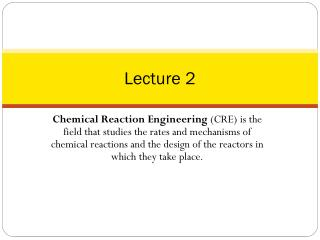# Lecture 2 - PowerPoint PPT PresentationDownload PresentationLecture 2

Lecture 2Download Presentation## Lecture 2

- - - - - - - - - - - - - - - - - - - - - - - - - - - E N D - - - - - - - - - - - - - - - - - - - - - - - - - - -
##### Presentation Transcript

1. Lecture 2 Chemical Reaction Engineering (CRE) is the field that studies the rates and mechanisms of chemical reactions and the design of the reactors in which they take place.

2. Lecture 2 – Tuesday 1/15/2013 • Review of Lecture 1 • Definition of Conversion, X • Develop the Design Equations in terms of X • Size CSTRs and PFRs given –rA= f(X) • Conversion for Reactors in Series • Review the Fall of the Tower of CRE

3. Review Lecture 1 ReactorMole Balances Summary NA FA FA The GMBE applied to the four major reactortypes (and the general reaction AB) t W V Batch CSTR PFR PBR

4. Review Lecture 1 CSTR – Example Problem Given the following information, Find V Liquidphase

5. Review Lecture 1 CSTR – Example Problem (1) Mole Balance: (2) Rate Law: (3) Stoichiometry:

6. Review Lecture 1 CSTR – Example Problem (4) Combine: (5) Evaluate:

7. Define conversion, X Consider the generic reaction: Chose limiting reactant A as basis of calculation: Define conversion, X

8. Batch

9. Batch Integrating, The necessary t to achieve conversion X.

10. CSTR Consider the generic reaction: Chose limiting reactant A as basis of calculation: Define conversion, X

11. CSTR Well Mixed Steady State

12. CSTR CSTR volumenecessary to achieveconversion X.

14. PFR Integrating, PFR volume necessary to achieve conversion X.

15. ReactorMole Balances Summary in terms of conversion, X X Batch t CSTR X W PFR PBR

16. Levenspiel Plots ReactorSizing Given –rA as a function of conversion, -rA= f(X), one can size any type of reactor. Wedo this by constructing a Levenspielplot. Here we ploteither (FA0/-rA) or (1/-rA) as a function of X. For (FA0/-rA) vs. X, the volume of a CSTR and the volume of a PFR can be represented as the shaded areas in the Levenspiel Plots shown as:

17. Levenspiel Plots

18. Area = Volume of CSTR F 0 A - r A X 1 CSTR

19. PFR

20. LevenspielPlots

21. Numerical Evaluations of Integrals • The integral to calculate the PFR volume can be evaluated using method as Simpson’s One-Third Rule: (See Appendix A.4) Other numerical methods are: • Trapezoidal Rule (uses two data points) • Simpson’s Three-Eight’s Rule (uses four data points) • Five-Point Quadrature Formula

22. Reactors in Series Given: rA as a function of conversion, one can also design any sequence of reactors in series by defining X: Only valid ifthere are no sidestreams. Molar Flow rate of species A at point i:

23. Reactors in Series

24. Reactors in Series Reactor 1: V1

25. Reactors in Series Reactor 2: V2

26. Reactors in Series Reactor 3: V3

27. Reactors in Series

28. Reactors in Series Space time τ is the time necessary to process 1 reactor volume of fluid at entrance conditions.

29. KEEPING UP • The tower of CRE, is it stable?

30. Reaction Engineering Mole Balance Rate Laws Stoichiometry These topics build upon one another.

31. Heat Effects Isothermal Design Stoichiometry Rate Laws Mole Balance CRE Algorithm

32. Rate Laws Mole Balance Be careful not to cut corners on any of the CRE building blocks while learning this material!

33. Isothermal Design Heat Effects Rate Laws Stoichiometry Mole Balance Otherwise, your Algorithm becomes unstable.

34. End of Lecture 2